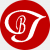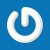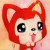﻿ 详解php中的闭包 - 小周博客,小周个人博客,程序猿小王子,技术博客,个人博客模板,php博客系统,设计模式,wzyl - 黑夜遮不住光亮

# 详解php中的闭包

php5.3有一个非常赞的新特性，那就是支持匿名函数(闭包)。匿名函数可用于动态创建函数，并保存到一个变量中。

```\$func = function()
{
exit('Hello world!!');
}; //这里必须要有;结尾

\$func();```

```function func()
{
exit('Hello world!!');
}

func();```

```function operate(\$operator)
{
if(\$operator == "-")
{
return function(\$a,\$b)
{
return \$a-\$b;
};
}
else
{
return function(\$a,\$b)
{
return \$a+\$b;
};
}
}

\$subtraction = operate("-");
echo \$subtraction(4,3); //1

```<?php
class Di
{
private \$_factory;

public function set(\$id,\$value)
{
\$this->_factory[\$id] = \$value;
}

public function get(\$id)
{
\$value = \$this->_factory[\$id];
return \$value();
}
}

class User
{

{
}

{
}
}

//从这里开始看
\$di = new Di();
\$di->set("zhangsan",function(){
return new User('张三');
});

\$di->set("lisi",function(){
return new User("李四");
});

```function func1(\$a)
{
return function() use (\$a)
{
echo \$a;
};
}

\$a = func1("a");

\$a();//输出a```

```\$variable = 10;

\$callback = function() use(\$variable){

echo ++\$variable."<br/>";
};

\$callback();  //输出：11

echo \$variable; //输出：10```

```\$variable = 10;

\$callback = function() use(&\$variable){

echo ++\$variable."<br/>"; //注意：我们这里是用的引用(&)方式
};

\$callback();  //输出：11

echo \$variable; //输出：11```### 精彩评论

1.1楼

好牛，已测试

2016-02-28 12:04:01
2.2楼
naive

浏览器换了无数个都看不到密码

2017-02-23 04:06:13
•@naive 现在已被屏蔽。我再放上去，我的网站就被屏蔽咯

2017-02-24 01:46:37